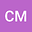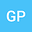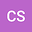loading page

Neimark-Sacker, flip and transcritical bifurcation in a symmetric system of difference equations with exponential terms
•••• Chrysoula Mylona,
• Garyfalos Papaschinopoulos,
• Christos Schinas
Chrysoula Mylona
Democritus University of Thrace
Author ProfileGaryfalos Papaschinopoulos
Democritus University of Thrace
Author ProfileChristos Schinas
Democritus Univ. of Thrace
Author Profile## Abstract

In this paper, we study the conditions under which the following symmetric system of difference equations with exponential terms: $x_{n+1} =a_1\frac{y_n}{b_1+y_n} +c_1\frac{x_ne^{k_1-d_1x_n}}{1+e^{k_1-d_1x_n}},$ $y_{n+1} =a_2\frac{x_n}{b_2+x_n} +c_2\frac{y_ne^{k_2-d_2y_n}}{1+e^{k_2-d_2y_n}}$ where $a_i$, $b_i$, $c_i$, $d_i$, $k_i$, for $i=1,2$, are real constants and the initial values $x_0$, $y_0$ are real numbers, undergoes Neimark-Sacker, flip and transcritical bifurcation. The analysis is conducted applying center manifold theory and the normal form bifurcation analysis.

#### Peer review status:ACCEPTED

09 Feb 2021Submitted to Mathematical Methods in the Applied Sciences
10 Feb 2021Submission Checks Completed
10 Feb 2021Assigned to Editor
15 Feb 2021Reviewer(s) Assigned
28 Feb 2021Review(s) Completed, Editorial Evaluation Pending
01 Mar 2021Editorial Decision: Revise Minor
03 Mar 20211st Revision Received
04 Mar 2021Submission Checks Completed
04 Mar 2021Assigned to Editor
05 Mar 2021Reviewer(s) Assigned
05 Mar 2021Review(s) Completed, Editorial Evaluation Pending
07 Mar 2021Editorial Decision: Accept